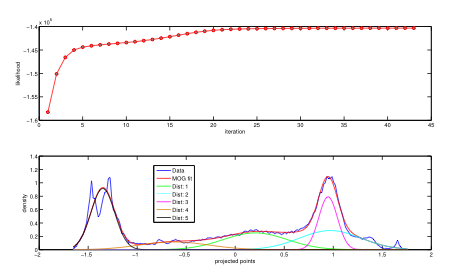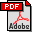## PMOG: The projected mixture of Gaussians model with application to blind source separation

### Abstract

We extend the mixtures of Gaussians (MOG) model to the projected mixture of Gaussians (PMOG) model. In the PMOG model, we assume that q dimensional input data points z_i are projected by a q dimensional vector w into 1-D variables u_i. The projected variables u_i are assumed to follow a 1-D MOG model. In the PMOG model, we maximize the likelihood of observing u_i to find both the model parameters for the 1-D MOG as well as the projection vector w. First, we derive an EM algorithm for estimating the PMOG model. Next, we show how the PMOG model can be applied to the problem of blind source separation (BSS). In contrast to conventional BSS where an objective function based on an approximation to differential entropy is minimized, PMOG based BSS simply minimizes the differential entropy of projected sources by fitting a flexible MOG model in the projected 1-D space while simultaneously optimizing the projection vector w. The advantage of PMOG over conventional BSS algorithms is the more flexible fitting of non-Gaussian source densities without assuming near-Gaussianity (as in conventional BSS) and still retaining computational feasibility.

### Core idea

1. In a seminal paper, Hyvarinen et al. (1998) developed approximations to the differential entropy of a random variable using a maximum entropy distribution (MED) approach. This MED distribution is assumed by Hyvarinen et al. (1998) to be "not very far from a Gaussian distribution". The theory developed in this paper is central to the operation of several ICA algorithms such as FastICA (FICA).

2. In another seminal paper, Attias et al. (1999) developed a general solution to the BSS problem where the latent source density was modeled as a "factorial MOG" density. This significant advance removed the "near Gaussianity" assumption on latent source densities. In addition, Attias et al. (1999) developed an expectation maximization (EM) algorithm for the exact maximum likelihood (ML) solution of the BSS problem.

3. However the exact ML approach of Attias et al. (1999) becomes computationally intractable for >13 latent sources and one has to resort to approximate variational inference. In addition, the approach of Attias et al. assumes "exact independence" between latent sources, i.e., does not allow partial dependence between sources.

4. The present work is mainly inspired by the work of Hyvarinen et al. (1998) and Attias et al. (1999). The main contributions of this work are:

1. Development of the PMOG model including an EM algorithm for its estimation

2. Removing the "near-Gaussianity" assumption in negentropy approximations of Hyvarinen et al. (1998) via the PMOG model

3. Retaining computational feasibility for >13 sources

4. Allowing partial dependence betweeen latent sources

### Key references

1. Gautam V. Pendse, "PMOG: The projected mixture of Gaussians model with application to blind source separation", arXiv:1008.2743v1 [stat.ML, cs.AI, stat.ME], 46 pages, 9 figures, 2010. [5.5 MB] (submitted) [download pdf] (this work)

2. A. Hyvarinen, "New approximations of differential entropy for independent component analysis and projection pursuit". Advances in Neural Information Processing Systems, 10:273-279, 1998. [168 KB] [download pdf] (related work)

3. H. Attias. "Independent factor analysis". Neural Computation, 11:803-851, 1999. [647 KB] [download pdf] (related work)

### Download Code

Matlab code for estimating the PMOG model and performing PMOG based BSS can currently be obtained under the terms of the license described below by sending an e-mail to gautam dot pendse at gmail.com. This code will be made freely available in the near future. Code distribution includes the following directories:

#### webpage

This directory contains a standalone version of this webpage pmog.html for offline browsing.

#### code

This directory contains code for:
1. estimating a mixture of PPCA model ppca_mm.m

2. estimating a PMOG model projected_mog_ica.m

3. doing orthogonal and non-orthogonal PMOG based BSS ppca_mm_ica.m and ppca_mm_ica_no_orth.m

4. running simulations on synthetic data similar to that in the paper create_mog_source_mixture.m, projected_mog_ica_test.m

5. component matching across multiple runs of BSS raicar_type_sorting_mat.m

6. running the 3 demos (see below), pmog_demo1.m, pmog_demo2.m and pmog_demo3.m

#### data

This directory contains the following data:
1. Natural image data used in the paper can be found in image_pmog_data1.mat and image_pmog_data2.mat. Each image was individually pre-processed by making it zero mean and unit standard deviation prior to further processing. Here's a step by step example of pre-processing. Code for pre-processing natural images from BSD preprocess_image.m is included. Here's a readme file for image data.

2. Synthetic data can be found in synthetic_pmog_data.mat. Here's a readme file for synthetic data.

#### demos

This directory contains the results of running pmog_demo1.m, pmog_demo2.m and pmog_demo3.m that have been saved as html files:
1. pmog_demo1.m illustrates the application of PMOG based BSS to a synthetic mixture generated using the FastICA package based on the work of Hyvarinen et al. (1998). Here's a webpage containing the step by step procedure and results of running pmog_demo1.m.

2. pmog_demo2.m illustrates the application of PMOG based BSS to a synthetic mixture generated using MOG source densities using the included code create_mog_source_mixture.m. Here's a webpage containing the step by step procedure and results of running pmog_demo2.m.

3. pmog_demo3.m illustrates the application of PMOG based BSS to natural image data from the Berkeley Segmentation Dataset and Benchmark (BSD): http://www.eecs.berkeley.edu/Research/Projects/CS/vision/grouping/segbench/. This data is also included in the above zip file (in a Matlab .mat file called image_pmog_data2.mat).

In pmog_demo3.m we use non-orthogonal PMOG to account for the fact that the sources are in fact not exactly independent. Here's a webpage containing the step by step procedure and results of running pmog_demo3.m. This demo essentially regenerates results shown in Figure7 [BSS performance for natural image dataset 2] from the paper.

#### License

Software for PMOG based BSS by Gautam V. Pendse is licensed under the terms described in the file license.html. Permissions beyond the scope of this license may be available at gautam dot pendse at gmail dot com.

Copyright © 2010, Gautam V. Pendse
e-mail: gpendse@mclean.harvard.edu